# 城市道路交通控制子区划分策略研究Study on Sub-Area Division Strategy of Urban Road Traffic Control

• 全文下载: PDF(1408KB)    PP.351-358   DOI: 10.12677/OJTT.2018.75043
• 下载量: 245  浏览量: 754   科研立项经费支持

Because of the complexity, randomness and dynamics of the urban road system, it is obviously unrealistic to unify the traffic guidance and control of the whole road network. Therefore, in order to achieve effective traffic guidance and control, the whole traffic network system should be zoned according to the development of urban space and function. The paper expounds the present research situation of traffic control sub-area division by static and dynamic methods and points out the shortcomings, gives the principle of dividing the traffic control sub-area according to the node state, and designs traffic control cell partition algorithm in detail. Finally, the standard test network Sioux Falls network is used to verify the partitioning algorithm. Case analysis shows that the algorithm can achieve effective traffic guidance and control, and alleviate traffic congestion.

1. 引言

1971年，美国学者Walinchus首次给出交通控制小区的定义  。Yagoda等学者从数学建模的角度考虑，对子区划分的控制指标、控制阈值和划分算法进行了深入研究，但最后并没有得出具体的子区划分方法  。由于早期的子区划分方法一般使用静态方法划分，以路网的物理特征作为划分依据，所以不能适应目前复杂的动态交通变化需求。为了找到与目前复杂交通系统中交通诱导与控制相匹配的子区划分方法，于是开始着眼于动态角度研究。王学堂把区域复杂系统分解为几个简单系统，对各个子系统进行研究  。高云峰、杨晓光等主要考虑物理关联和路径关联等原则，对交叉口群的划分方法进行了研究，把交叉口间的关联性作为划分交叉口群的依据  。这些子区划分方法的是基于常态条件下的交通控制，对非常态交通条件下的交通控制子区的划分方法并没有深入研究。除此以外，王薇还从诱导角度出发，讨论了基于网络平衡的大范围交通流动态管理，她提到为解决计算效率瓶颈问题，应对路网进行有效划分，提出了在路网诱导层面划分诱导子区的解决思路  。

2. 交通控制子区划分原则

1) 中心关键–周边疏通原则

2) 拥挤低谷点作边界原则

3) 交通状态相似原则

4) 距离范围为上限原则

3. 交通控制子区划分算法

Step 1：初始化：建立集合M，元素为所有路段交叉口节点；建立若干集合Mi，设置为空集，算法结束后Mi为第i个小区的节点集合；设s = 0；

Step 2：计算所有节点 $j\left(j=1\cdots m,\text{}j\in N\right)$ 的可达值 $Q\left(i\right)$ ，所有链路 ${l}_{ij}$ 的路段交通状态系数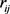Step 3：新建集合T，将所有 $Q\left(i\right)$ 按照从小到大排序，并依序置入集合T；

Step 4：从T中依序取出 $Q\left(i\right)$ ，进行以下操作：

1) 判断是否满足 ${C}_{ij}\left({\lambda }_{\tau }\right)=0$${c}_{ij}\left({\lambda }_{\tau }\right)=0$ ，若不满足，结束Step 4，否则转(2)；

2) 判断是否满足 $i\in M,j\in M$ ，若不满足，转(3)，否则：节点 $i$$j$ 置入新建集合 $Ms$ 中，同时从集合M中删除，即 $s=s+1,Ms=\left\{i,j\right\},M=M-\left\{i,j\right\}$ ；并结束Step 4；

3) 判断是否满足 $i\in M,j\in Mq$ ，若满足，转(4)，否则，转(6)；

4) 判断若节点i加入到结合 $Mq$ ，则 $Mq$ 最大跨距是否大于1000米，若是，则转(5)，否则结束Step 4；

5) 判断是否满足 ${p}_{i}>{p}_{k},\forall k\in Mq$ ，若满足，则节点i加入到集合 $Mq$ ，集合M中删除节点i；否则， $s=s+1,Ms=\left\{i,j\right\},Mq=Mq-\left\{j\right\}$ ；完成后结束Step 4；

6) 判断是否满足 $i\in {M}_{a},j\in {M}_{b}$ ，若是，转(7)，否则，结束Step 4；

7) 判断若 ${M}_{a}$${M}_{b}$ 合并，合并后最大跨距是否小于1000米，若是则转(8)，否则结束Step 4；

8) 判断对任意 $x\in {M}_{a},y\in {M}_{b}$ ，是否有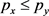成立，若是，则 ${M}_{a}={M}_{a}\cup {M}_{b}$ ，结束Step 4；否则，转(9)；

9) 排序比较，确定 ${M}_{a}$ 中关键路口 ${c}_{a}$${M}_{b}$ 中关键路口 ${c}_{b}$ ；判断 ${c}_{a}$${c}_{b}$ 的距离是否小于1000米，若是则转(10)，否则结束Step 4；

10) 判断是否满足 ${p}_{a}<{p}_{b}$ ，若是，则 ${M}_{b}={M}_{a}\cup {M}_{b}$ ，否则 ${M}_{a}={M}_{a}\cup {M}_{b}$

Step 5：判断集合T是否清空，若是，转Step 6，否则转Step 4；

Step 6：对M中剩余的每个元素i，进行操作：新建集合 ${M}_{s}$ ，将i置入 ${M}_{s}$ ，同时从M中删除节点i，即 $s=s+1,{M}_{s}=\left\{i\right\},M=M-\left\{i\right\}$

Step 7：算法结束。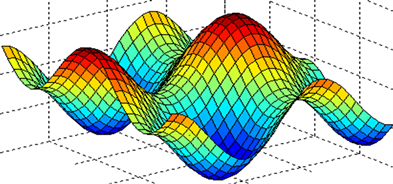Figure 1. Graph of peak-trough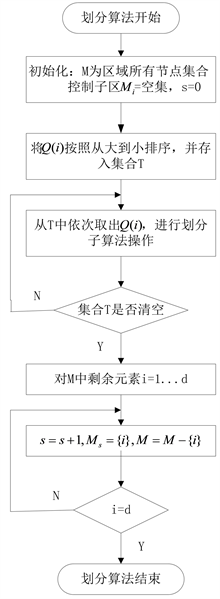Figure 2. Control subarea division main algorithm flow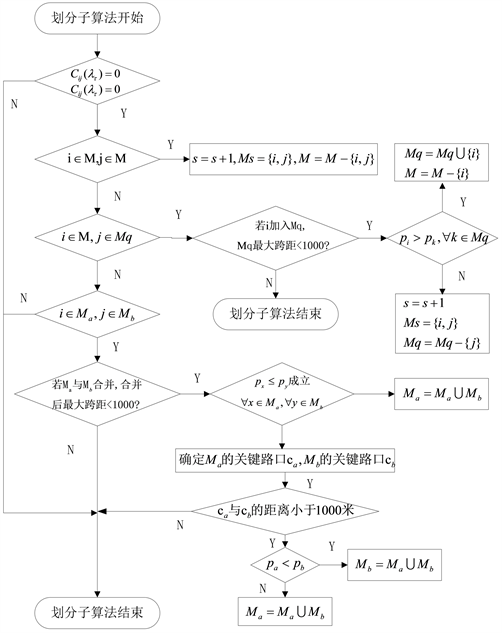Figure 3. Control subarea division sub-algorithms flow

4. 实例分析

${t}_{a}\left(x\right)={t}_{a0}\left[1+\alpha {\left(\frac{{x}_{a}}{{C}_{a}}\right)}^{\beta }\right]$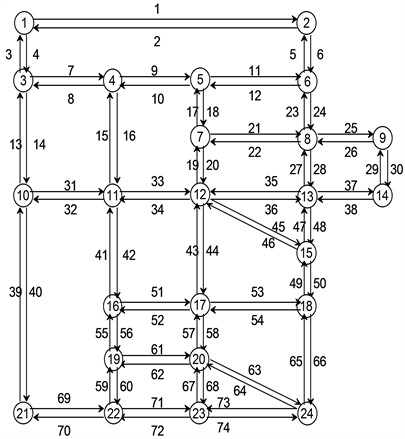Figure 4. An example of a road network based on Sioux Falls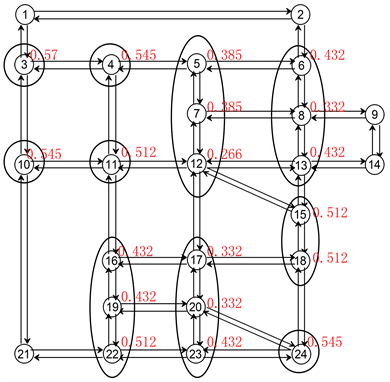Figure 5. Traffic control subarea division with a saturation of 0.6Table 1. Traffic requirements under different conditionsTable 2. Correlation parameter values of sections in Sioux Falls networkFigure 6. Traffic control subarea division with a saturation of 1.0

5. 结论

NOTES

*通讯作者。

  Walinchus, R.J. (1971) Real-Time Network Decomposition and Sub-Network Interfacing. Highway Research Record, No. 366, 20-28.  Yagoda, H.N., Principle, E.H., Vick, C.E. and Leonard, B. (1973) Subdivision of Signal Systems into Control Areas. Traffic Engineering, 43, 42-45.  王学堂. 城市交通信号整体优化理论的概念与方法[J]. 长安大学学报(自然科学版), 2002, 22(5): 92-94.  胡华, 高云峰, 杨晓光. 考虑路网OD路径的交叉口群动态划分方法[J]. 计算机工程与应用, 2010, 46(31): 1-4+18.  王薇. 基于网络平衡的大范围交通协调控制系统理论及技术研究[D]: [博士学位论文]. 长春: 吉林大学, 2008.  陈珊珊. 城市道路区域交通信号控制的动态子区划分[D]: [硕士学位论文]. 南京: 东南大学, 2016.